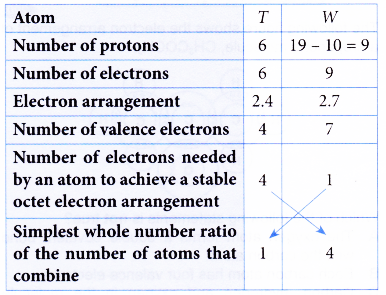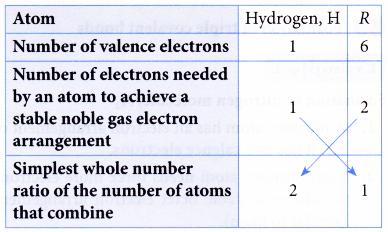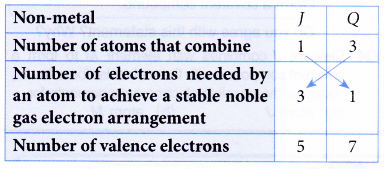## Describe how to write a formula for a covalent compound

Method to deduce the formulae of covalent compounds

Non-metals combine with non-metals to form covalent compounds.
Table shows the number of electrons needed by an atom of a non-metal to achieve a stable noble gas electron arrangement. The number of electrons needed is a measure of the combining power of the non-metal.

 Element Number of valence electrons Number of electrons needed to achieve a stable noble gas electron arrangement Group 17 7 1 Group 16 6 2 Group 15 5 3 Carbon and Silicon in Group 14 4 4 Hydrogen 1 1

The molecular formulae of covalent molecules formed from non-metals of Group 14, Group 15, Group 16, Group 17 and hydrogen can be deduced as shown in Table.

 Non-metals Molecular formula X Y Group 14 Group 17 XY4 Group 14 Group 16 XY2 Group 15 Group 17 XY3 Group 16 Group 17 XY2 Hydrogen Group 17 XY (HY) Hydrogen Group 16 X2Y( H2Y) Group 15 Hydrogen XY3 (XH3)

The information in above Tables can be used to determine the formulae of covalent compounds as shown in the examples below.

## Writing formulas for covalent compounds examples

1. Atom T has a proton number of 6. Atom W has 10 neutrons and a nucleon number of 19. Atoms T and W combine to form a compound. Determine the molecular formula of the compound.
Solution:One atom of T combines with 4 atoms of W to form a compound.
∴ Molecular formula = TW4

2. Element R is located in Group 16 and Period 3 of the Periodic Table. Element R reacts with hydrogen to form a compound. Determine the molecular formula of the compound formed. (Proton number : H = 1)
Solution:
Atom R has 6 valence electrons because it is situated in Group 16 of the Periodic Table.
Proton number of a hydrogen atom = 1
∴ Electron arrangement of a hydrogen atom = 1.
∴ A hydrogen atom has one valence electron.Two hydrogen atoms combine with one R atom
∴ Molecular formula = H2R

3. Non-metal J reacts with non-metal Q to form a molecule with the molecular formula JQ3. Which groups of the Periodic Table do J and Q belong to?
Solution:
Simplest whole number ratio of the number of atoms of J : Q = 1 : 3.Hence, J is from Group 15 because it has 5 valence electrons.
Q is from Group 17 because it has 7 valence electrons.

## What is the difference between ionic and covalent bonds?

Comparison between the formation of ionic and covalent bonds:

The structural changes during the formation of ionic and covalent bonds from their respective atoms are different.
Table compares and contrasts the structural changes during the formation of ionic and covalent bonds.

 Ionic bond Covalent bond 1. It is formed when metal atoms combine with non­metal atoms. 1. It is formed when non­metal atoms combine with non-metal atoms. 2. It involves the transfer of electrons from metal atoms to non-metal atoms. 2. It involves the sharing of electrons between atoms of non-metals. 3. Metal atoms lose electrons to form positive ions, whereas non-metal atoms gain electrons to form negative ions. 3. Each non-metal atom contributes an equal number of electrons to each other for sharing. 4. Formation of positive and negative ions enables the ions to achieve stable noble gas electron arrangements. 4. Two atoms share 1,2 or 3 pairs of electrons to enable each atom to achieve a stable noble gas electron arrangement. 5. Oppositely-charged ions are held together by strong electrostatic forces of attraction, Known as ionic bonds. 5. Atoms in a molecule are bonded together by covalent bonds, whereas molecules are held together by weak forces of attraction.